# Titration pH Curves

This is part of the HSC Chemistry course under the topic Quantitative Analysis.

### HSC Chemistry Syllabus

• investigate titration curves and conductivity graphs to analyse data to indicate characteristic reaction profiles, for example:
– strong acid/strong base
– strong acid/weak base
– weak acid/strong base (ACSCH080, ACSCH102)

### Equivalence Point and Endpoint in Titration

• Equivalence point is the point at which acid and base react in their stoichiometric ratio to reach complete neutralisation.

There are no acids and bases in excess when a titration reaches its equivalence point. It is important to keep in mind that the stoichiometric ratio between an acid and a base is not always 1 to 1.

$$1:1 \hspace{0.2cm} ratio: NaOH + HCl → NaCl + H_2O$$

$$2:1 \hspace{0.2cm} ratio: 2NaOH + H_2SO_4 → Na_2SO_4 + 2H_2O$$

$$3:1 \hspace{0.2cm} ratio: 3NaOH + H_3PO_4 → Na_3PO_4 + 3H_2O$$

• Endpoint: the point in the titration when the indicator just changes colour

A suitable indicator is one where its endpoint occurs at the same time as the equivalence point between the acid and base.

The pH of the final solution at equivalence point depends on the type of salt produced from neutralisation

• Neutral salt: pH = 7
• Acidic salt: pH < 7
• Basic salt: pH > 7

The type of salt formed depends on the relative strengths of acids and bases used in titration.

### Strong Acid and Strong Base Titration

• Produces neutral salt
• pH of resultant solution = 7 as the neutralisation always produces a neutral salt

Appropriate indicator(s) include

• Bromothymol blue (6.0-7.4)
• Phenolphthalein (8.3-10.0)
• Methyl orange (3.2-4.4)

An example of this titration is the reaction between hydrochloric acid and sodium hydroxide

$$NaOH(aq) + HCl(aq) → NaCl(aq) + H_2O(l)$$For a strong acid and strong base titration, there is a steep (almost vertical) pH change at equivalence point. This means at the time the titre volume is added, the equivalence point occurs simultaneously with endpoint of various indicators. Most indicators are suitable for strong acid-strong base titration.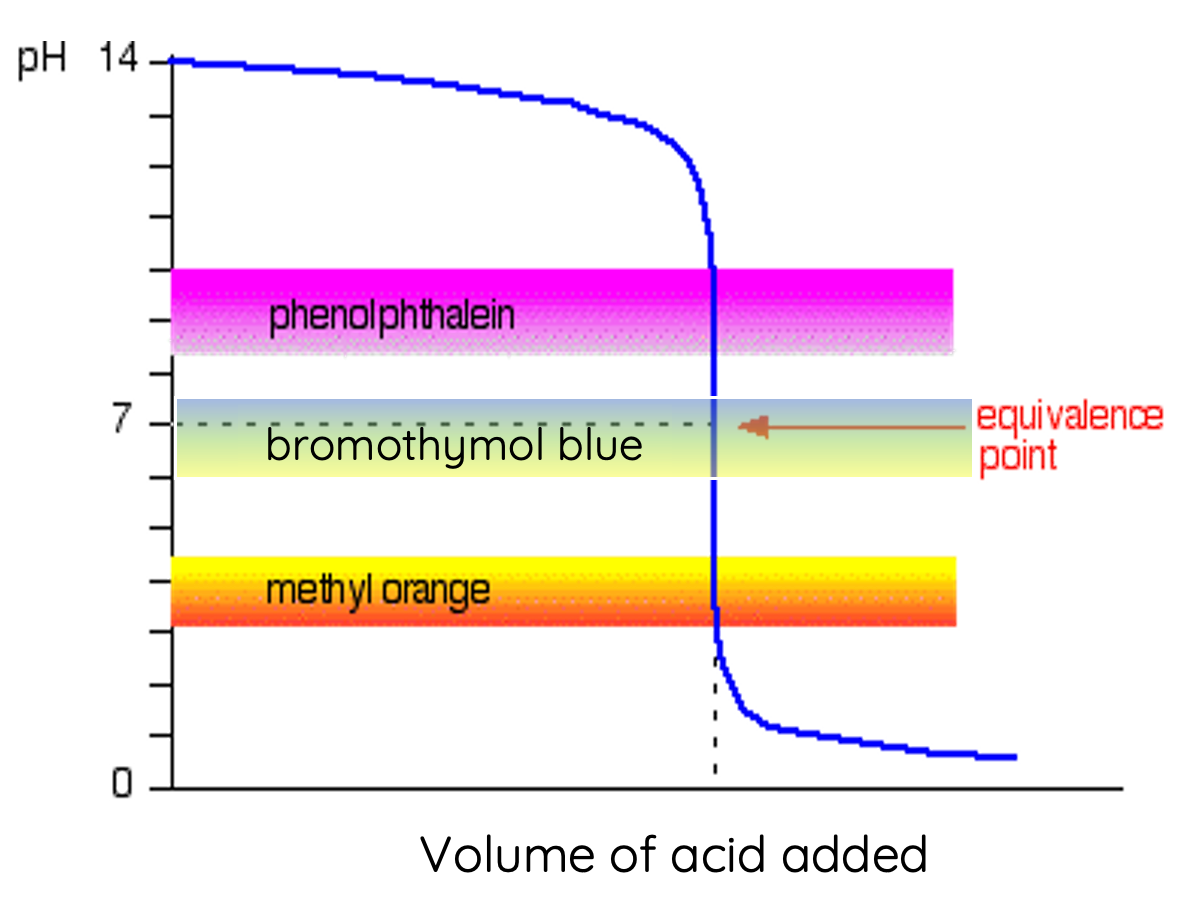### Strong Acid and Weak Base Titration

• Usually produces acidic salt
• Equivalent point at pH < 7
• Methyl orange (3.2-4.4) is an appropriate indicator
• Phenolphthalein (8.3-10.0) is NOT suitable

An example of a strong acid and weak base titration is the reaction between hydrochloric acid and ammonia.

$$HCl(aq) + NH_3(aq) \rightarrow NH_4Cl(aq)$$

The equivalence point of a strong acid and weak base titration is below pH of 7 due to the presence of an acidic salt.

Ammonium chloride is an acidic salt because ammonium ionises with water to form ammonia and hydronium ions

$$NH_4^+(aq) + H_2O(l) \rightleftharpoons NH_3(aq) + H_3O^+(aq)$$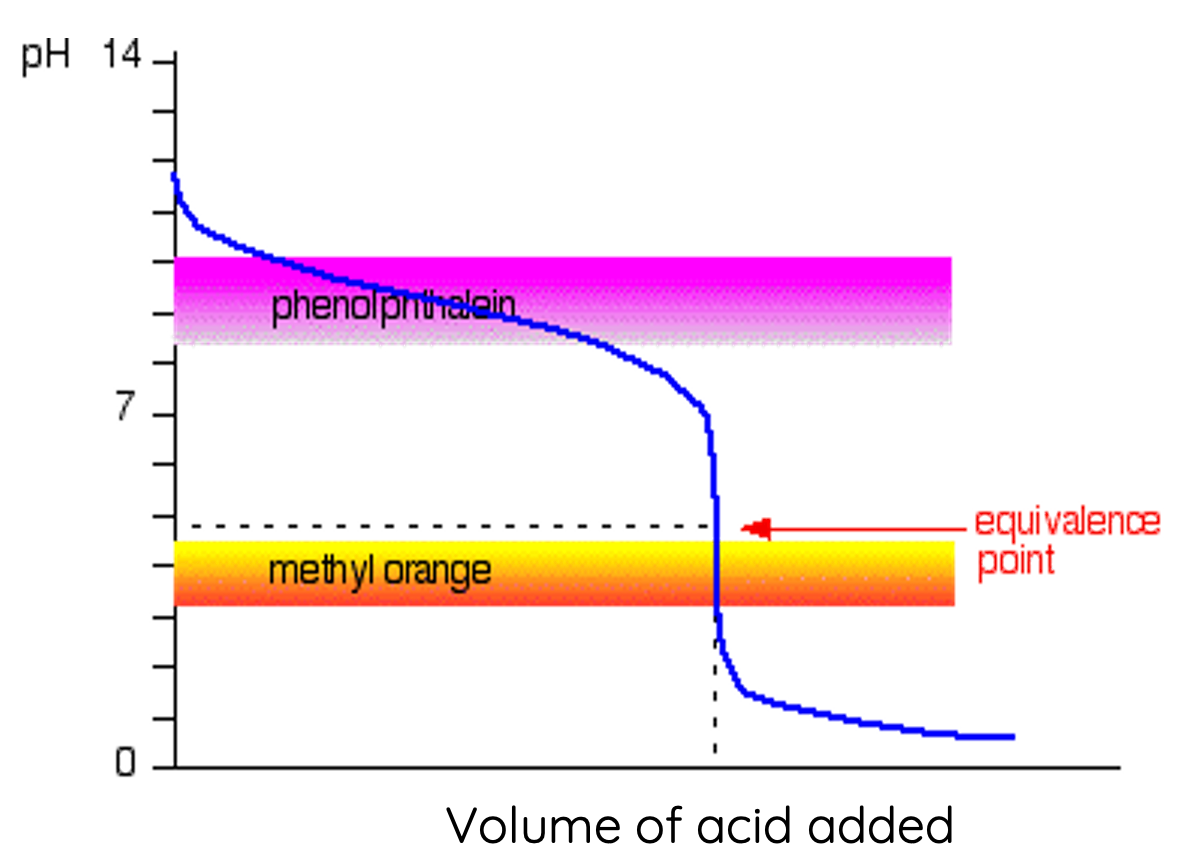As shown by the graph, the endpoint of phenolphthalein occurs much before the equivalence point, deeming it inappropriate. In contrast, when methyl orange is used as an indicator, the solution's colour will change from yellow to red at the same time as the equivalence point.

### Weak Acid and Strong Base Titration

• Produces basic salt
• pH of resultant solution > 7
• Phenolphthalein (8.3-10.0) is an appropriate indicator
• Methyl orange (3.2-4.4) is NOT suitable

An example of a weak acid and strong base titration is the reaction between acetic acid and sodium hydroxide

$$CH_3COOH(aq) + NaOH(aq) \rightarrow NaCH_3COO(aq) + H_2O(l)$$

The equivalence of a strong base and weak acid titration is above the pH of 7 due to the presence of a basic salt.

Sodium acetate is a basic salt because acetate ionises with water to form acetic acid and hydroxide ions

$$CH_3COO^–(aq) + H_2O(l) \rightleftharpoons CH_3COOH(aq) + OH^–(aq)$$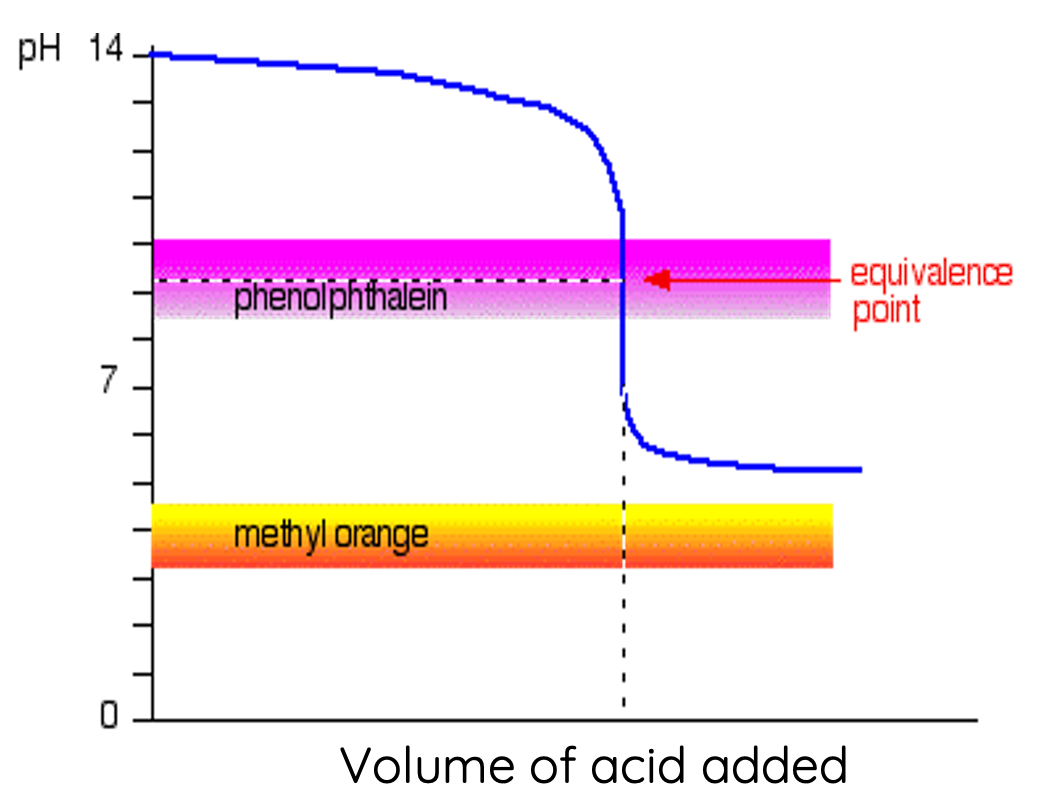As shown by the graph, methyl orange is not a suitable indicator for this titration because its endpoint occurs well after the equivalence point. Phenophthalein is a suitable indicator because the solution will decolourise from a pink colour at the same time as the equivalence.

### Weak Acid and Weak Base Titration

• Produces an acidic or basic salt
• pH of resultant solution varies
• If Ka of acid > Kb of base, pH < 7
• If Ka of acid < Kb of base, pH > 7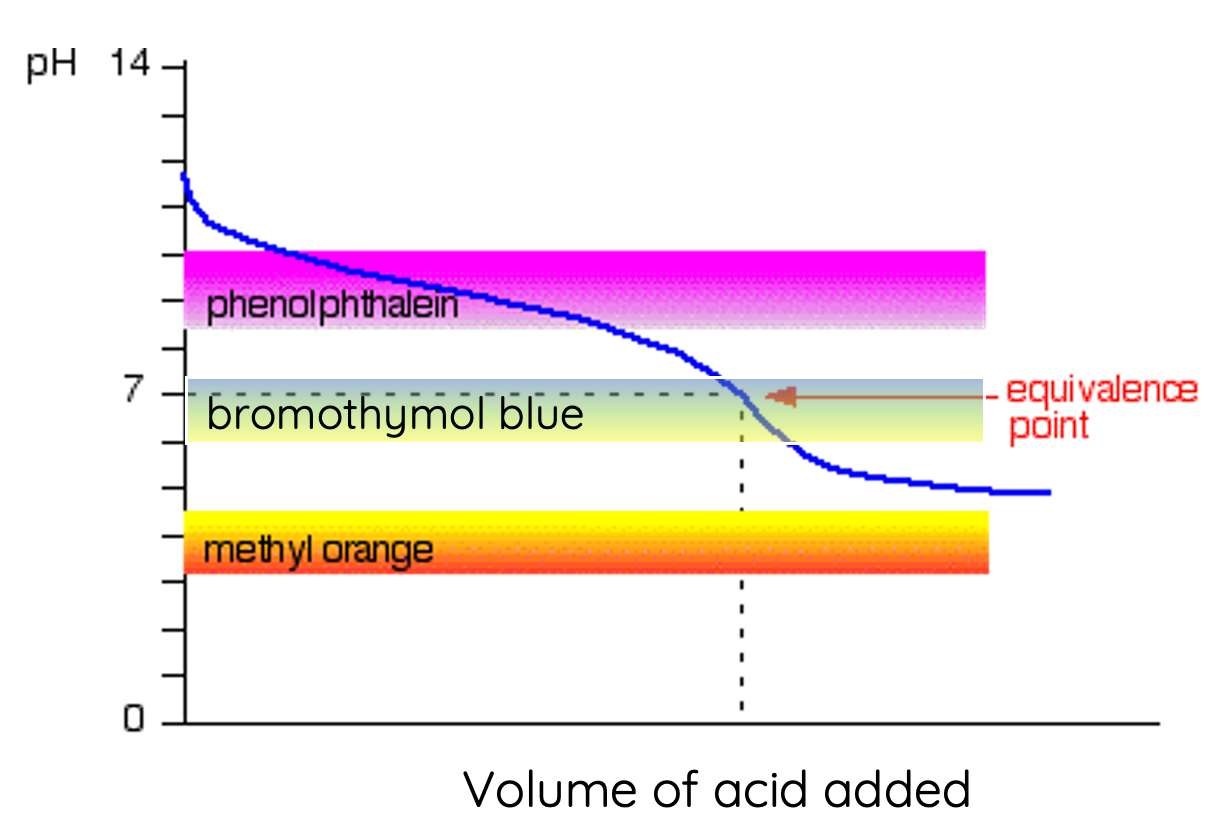The titration curve for weak acid and weak base titration has a characteristic flattened appearance. The ‘flatter’ change in pH is due to the formation of a buffer in the flask as titration takes place.

Direct titration involving weak acids and weak bases is generally avoided as there are not many suitable indicators. Direct titration in this case is often inaccurate as the equivalence point rarely coincides with the endpoint of indicators. Bromothymol blue (6.0-7.4) is most suitable indicator in the example shown.

### Titration Involving Polyprotic Acids

Polyprotic acids are acids with multiple acidic protons. Acidic protons are hydrogen atoms that can be deprotonated as hydrogen ions. During titration, each acidic proton has its own equivalence point.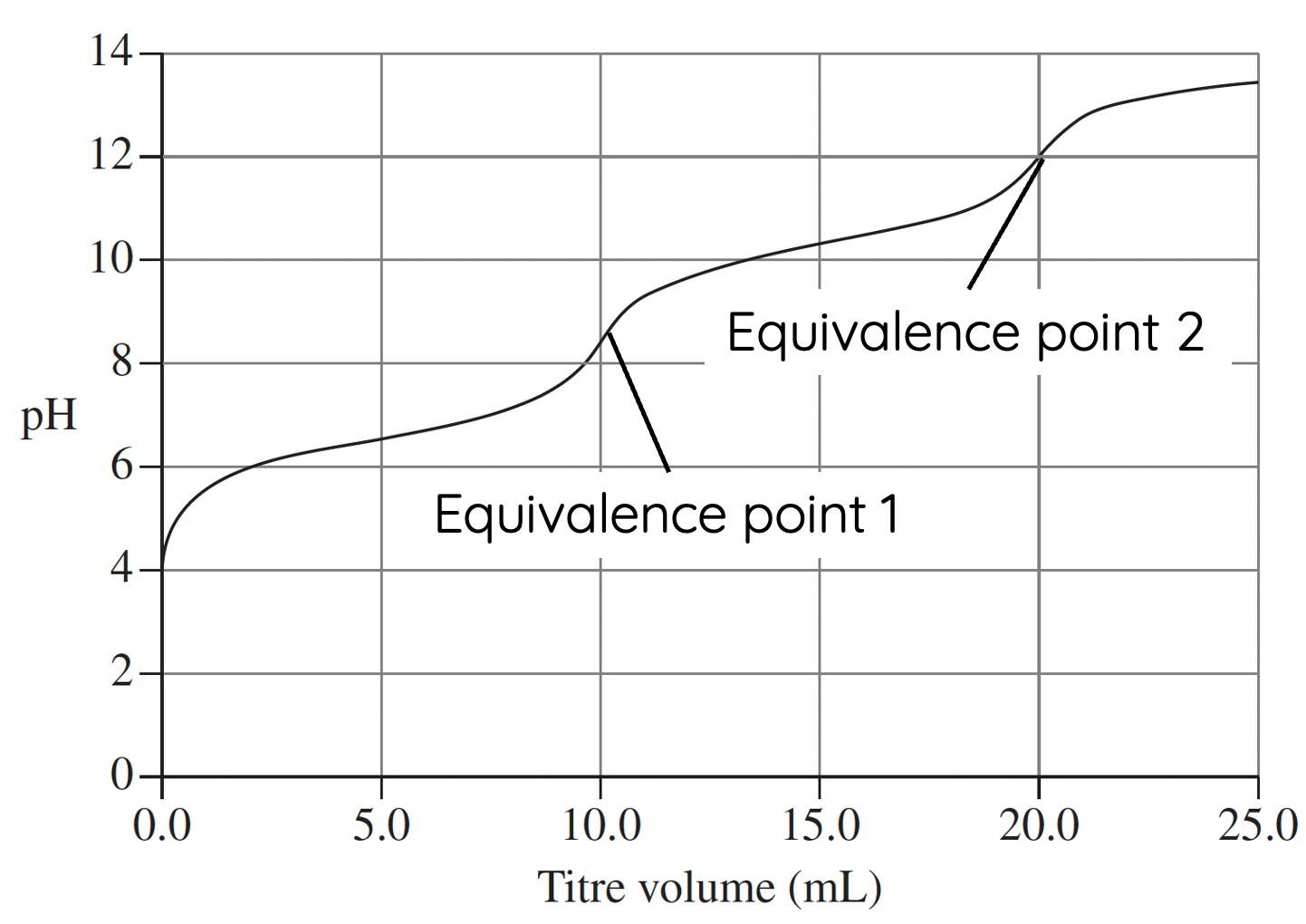The graph shows the titration curve for a weak diprotic acid e.g. carbonic acid.

### Buffer Region in Titration Curves

• A buffer is a system that will resist changes in pH whenever a small amount of acid or base is added to it.
• A buffer consists of comparable amounts of a weak acid and its conjugate base OR a weak base and its conjugate acid.

A buffer region can be seen in titration curves when the conical flask of the titration contains either a weak acid or a weak base.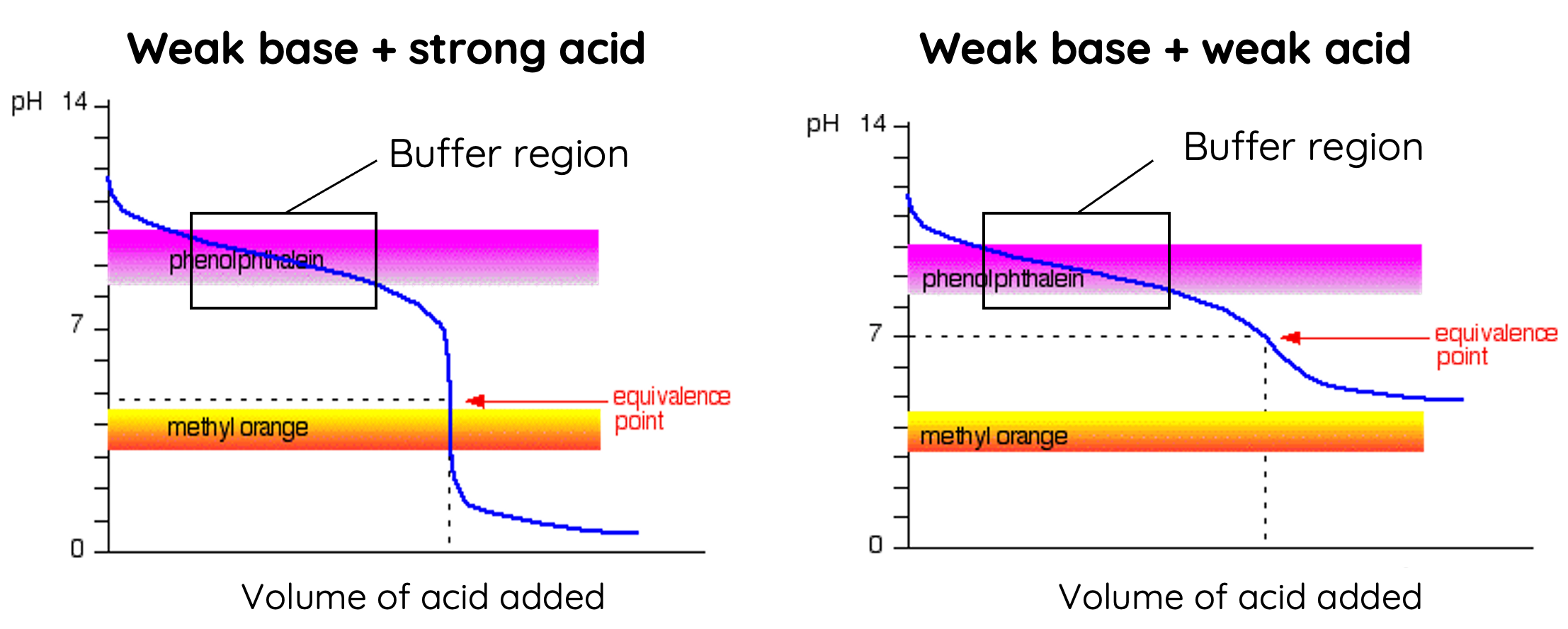The two titration curves shown above are produced when the conical flask contains a weak base. The pH of the solution starts above 7 as it is initially basic. The pH decreases as acid is added from the burette. However, the rate of decrease shortly decreases (flattens) due to the formation of a buffer. As acid is added, more base molecules gain protons to become conjugate acids, leading to a solution that consists of a comparable amount of weak base and its conjugate acid.

However, the rate of decrease in pH increases as more acid is added. This is because the number of conjugate acid molecules will eventually surpass the number of weak base left over in the conical flask. When the number of weak base molecules decreases, the capacity of the buffer to resist changes in pH when an acid is added decreases.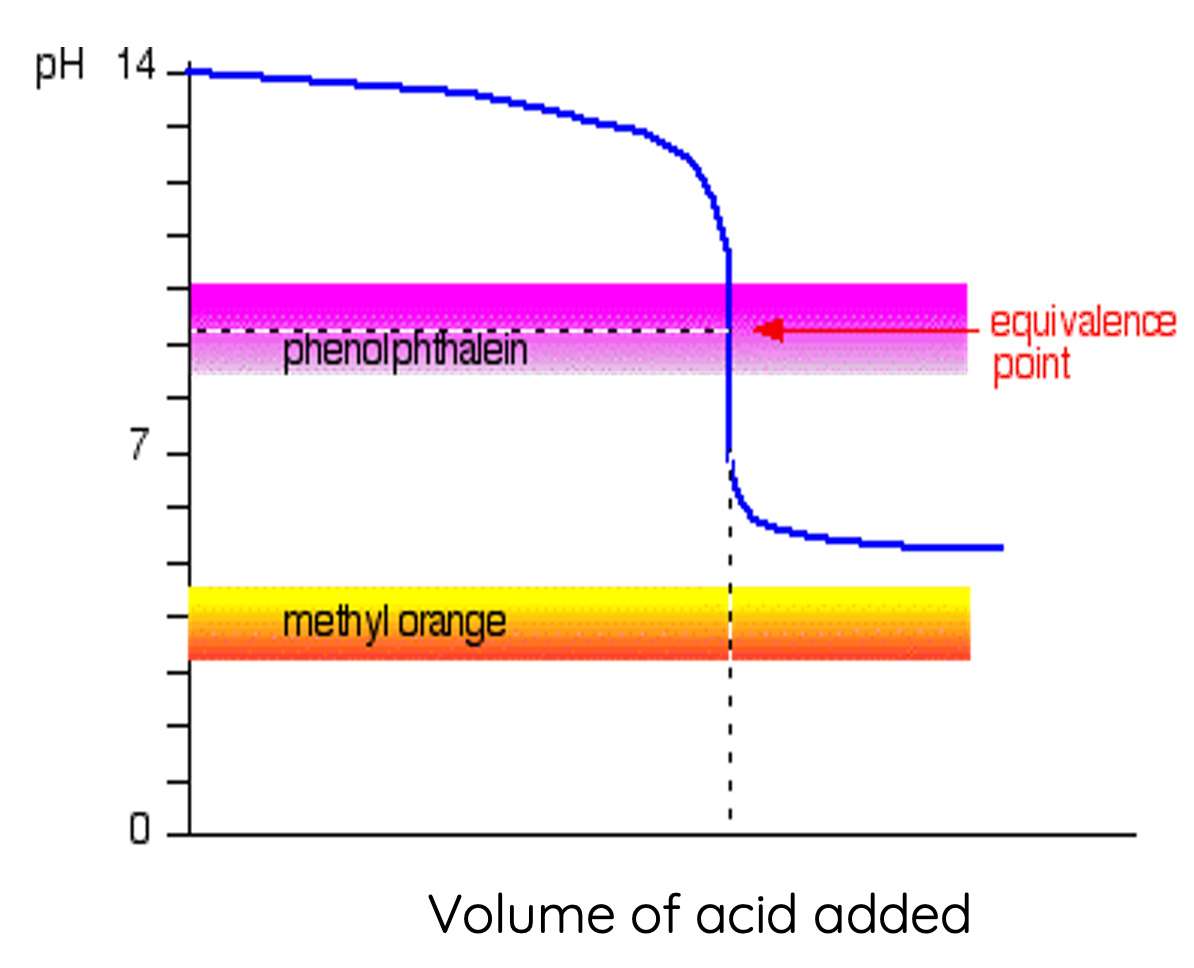The graph above shows a titration curve between a strong base (conical flask) and weak acid (burette). There is no buffer region present in this titration curve because a strong base is completely dissociated in solution. When a weak acid is added, it will react with hydroxide ions that are already present to form its conjugate base.

Since a buffer requires the presence of both the weak acid and its conjugate base, a buffer will never form in such a titration as any amount of weak acid added to the conical flask will quickly be neutralised by the hydroxide ions from the strong base.